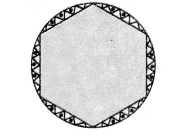# A round table cover has six equal designs as shown in the figure. If the radius

A round table cover has six equal designs as shown in the figure. If the radius of the cover is 28 cm, find the cost of making the designs at the rate of ₹0.35 per cm². (Use √3= 1.7)1 Like
1 Like